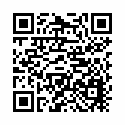## CCSS First grade math games / 1st grade math games

Rating: 0.00 (Votes: 0)

Searching for Common core first grade free learning app / CCSS 1st grade math games / grade 1 math games / first grade addition and subtraction worksheets.

You are at the right place. This first grade games app covers entire common core standard (CCSS). This math game for 1st grade has Infinite tests / worksheets . All System generated.

*** APP contains
► Word Problems for first graders
► Addition worksheets for 1st grade
► Subtraction worksheets for 1st grade
► Money
► Time identification for 1st grade
► Addition and Subtraction worksheets for 1st grade
► Place Value and Comparison worksheets for 1st grade
► Patterns worksheets for 1st grade
► Counting for 1st grade
► Measurement
► Geometry for 1\$st grade
Some tests are free and you have to upgrade to get unlimited access.

Common core math app has math activities / worksheets covers following common core standard syllabus :
1.OA - First grade math games - Operations and Algebraic Thinking
addition and subtraction worksheets for kids of 1st grade maths has :
► addition and subtraction within 20
► solve word problems - adding, subtracting
► Solve word problems - addition of three whole numbers
► solve Commutative property of addition
► solve problems related to properties of operations and the relationship between addition and subtraction.

addition and subtraction for kids first grade maths worksheets has test / quiz related to
► Add and subtract within 20
► Relate counting to addition and subtraction
► Work with addition and subtraction equations.

Common core math app has the following 2nd set of math activities / worksheets / test /quiz : 1.NBT Common core standard First grade math games -
Numbers and operations in Base Ten

► Count to 120, starting at any number less than 120.
► Read and write numerals and represent a number of objects with a written number
► Understand place value.
► two-digit number represent amounts of tens and ones.
► Compare two two-digit numbers based on meanings of the tens and ones digits.
► Use place value understanding and properties of operations to add and subtract.
► Add within 100, including adding a two-digit number and a one-digit number
► adding a two-digit number and a multiple of 10.
► place value, properties of operations, and/or the relationship between addition and subtraction
► Given a two-digit number, mentally find 10 more or 10 less than the number, without having to count;
► Subtract multiples of 10 in the range 10-90 from multiples of 10 in the range 10-90

Common core math app for 1st grade involves the following math activities :1.MD Common core standard First grade math games- Measurement and Data
► Measure lengths indirectly and by iterating length units
► Order three objects by length.
► Compare the lengths of two objects indirectly by using a third object.
► Express the length of an object as a whole number of length units.
► Scales used for measurement of length,weight etc
► Tell and write time. Problems involving identifying the time in the clock shown.

Next set of first grade math activities involves : 1.G Common core standard First grade math games- Geometry
► Reason with shapes and their attributes in first grade math geometry.
► defining attributes versus non-defining attributes
► first grade geometry math involving identification of e two-dimensional shapes (rectangles, squares, trapezoids,triangles, half-circles, and quarter-circles) or three-dimensional shapes (cubes, right rectangular prisms, right circular cones, and right circular
cylinders)
► first grade geometry involving identification of Partition circles and rectangles into two and four equal shares, describe the shares using the words halves, fourths, and quarters, and use the phrases half of, fourth of, and quarter of.

refer our other apps : First Grade math , Second Grade math, Third Grade math, Fourth Grade math, Fifth Grade math, Sixth Grade math, Multiplication tables.
User Reviews
Based on 0 Votes and 0 User Reviews
5 Star
0
4 Star
0
3 Star
0
2 Star
0
1 Star
0
Add Comment & Review
We'll never share your email with anyone else.
Tech News
Other Apps in This Category
Visit with QR Code• Category: Education
• Platform: Android
• Developer: Appraiser Kids Games
• File Size: 1.9M
• Visits: 0
• License: Free
• Last Update: February 02, 2021
Editor's Choice